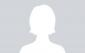# Bernoulis

4 posts / 0 new
Sydney SalesBernoulis

y' + y = e×

JanetAble

Hi,

I'll try)

(y'-y=0) are y=C(x)*exp(x) . Substitute in the equation with the right part, then we get : C'(x)*exp(x) =e^x, from c=x+a. So, y=(x+a)*e^x, where a= const....

------
Catalog of math sources by Professor M. Maheswaran:
http://uwc.edu/depts/math/resources/catalog

JanetAble

Woops... there were several ways to attack this type of problem here:

so now I don't know what is really right...

Veena ParmarThank you so much for your work,carry on,I have got very easy method for bernulli's equation

• Mathematics inside the configured delimiters is rendered by MathJax. The default math delimiters are $$...$$ and $...$ for displayed mathematics, and $...$ and $...$ for in-line mathematics.